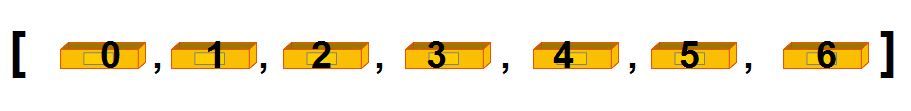# [GO] About the basics list of Python basics

This article is for Django Girls Japan Python beginners, This is a material for study sessions. In addition, since the author is also a beginner, we apologize for any problems.

# list

a = ["A","B","C","D","E","F","G"]

Suppose you have a list with 7 elements from A to G as above. The list has a room number.Counting from the left side, the first room number will be 0, so Let's be careful. When counting from the right, count as -1 -2 ....

## Make a list

### Write by hand

Just write it yourself. Enclose in [] and list the elements separated by commas. a = ["A","B","C","D","E","F","G"]

### List tuples

Substituting a tuple (this time taple) into a variable and making it a list (variable) will result in a list. taple = ("A","B","C","D","E","F","G") a = list(taple) a is a list of ["A", "B", "C", "D", "E", "F", "G"].

### List strings

a = list("ABCDEFG") a is a list of ["A", "B", "C", "D", "E", "F", "G"].

### Use split ()

i = "A,B,C,D,E,F,G" a = i.split(",") a is a list of ["A", "B", "C", "D", "E", "F", "G"]. split () splits by the character specified in the argument and makes a list.

For example ... If you try to put what is in i above as ↓ ... i = "AB,C,D,EF,G" a = i.split(",") a is a list of ["AB", "C", "D", "EF", "G"].

## Extraction using slices

If you specify the room number, you can retrieve the element. a →　"A"

By specifying the start position and end position, the specified range can be extracted. a[1:-3]　→ ["B", "C", "D"] a[1:4] → ["B", "C", "D"]

• These two are the same.

You can specify only the start position and then take out to the end. a[2:] → ["C","D","E","F","G"]

You can extract from the beginning to the specified position by specifying only the end position. a[:2] → ["A","B"]

## Add and remove values

### Add using + =

If you want to add an element to the end of an existing list, you can add it with + =. a = ["A","B","C","D","E","F","G"] a += "H" a is a list of ["A", "B", "C", "D", "E", "F", "G", "H"].

### Append using append ()

When you want to add an element to the end of an existing list List.append (what you want to add) You can add it with. a = ["A","B","C","D","E","F","G"] a.append("H") a is a list of ["A", "B", "C", "D", "E", "F", "G", "H"].

### Add using insert ()

You can add a value to any location by using insert (where you want to add, what you want to add). a = ["A","B","C","D","E","F","G"] a.insert(0, "W") a is a list of ["W", "A", "B", "C", "D", "E", "F", "G"].

### Remove using remove ()

It will be removed in the list .remove (what you want to remove), See from the left what you want to delete and delete only the first one.

a = ["A","B","C","D","E","F","G","A","B","C","D"] a.remove("B") a is a list of ["A", "C", "D", "E", "F", "G", "A", "B", "C", "D"].

### Delete using the room number (position) in the list

Delete it in the del list [room number]. a = ["A","B","C","D","E","F","G","A","B","C","D"] del a a is a list of ["A", "C", "D", "E", "F", "G", "A", "B", "C", "D"].

You can also delete by specifying the range of the list. a = ["A","B","C","D","E","F","G","A","B","C","D"] del a[2:4] a is a list of ["A", "B", "E", "F", "G", "A", "B", "C", "D"].

## Sorting

### Sorting using sort ()

Sorts the elements of the list in ascending order. a = [4, 6, 8, 1, 3] a.sort() a is a list of [1, 3, 4, 6, 8].

If you want to sort in descending order, specify reverse = True in the argument. a = [4, 6, 8, 1, 3] a.sort(reverse=True) a is a list of [8, 6, 4, 3, 1].

### Sorting using sorted ()

Sorts the elements of the list in ascending order without changing the original list. a = [4, 6, 8, 1, 3] b = sorted(a) b is a list of [1, 3, 4, 6, 8]. At this time, a is the original list of [4, 6, 8, 1, 3].

## Find out the number of elements

You can check the number of elements with len (list). a = ["A","B","C","D","E","F","G","A","B","C","D"] You can get 11 elements with len (a).